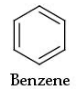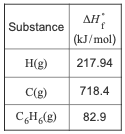# Problem: Part C. The average bond enthalpy for C − H is 413 kJ / mol. 413 kJ of energy is required to break a mole of CH into atoms:CH(g) → C(g) + H(g), ΔH = 413 kJUsing this information, and your answer from Part A, calculate the enthalpy change of the reaction from Part B. That is, calculate the energy required to break only the carbon-carbon bonds in benzene.Express your answer to four significant figures and include the appropriate units.Determine the standard enthalpy change for the reaction that represents breaking all of the bonds in benzene, determine the equation for breaking all the carbon-carbon bonds of benzene use Hess's law to calculate the enthalpy change of this reaction and calculate the average bond enthalpy of a carbon-carbon bond in benzene This tutorial has been developed from Brown/LeMay/Bursten Integrative Exercise 8.105 and is a multiple-concept tutorial.Consider benzene in the gas phase, C6H6(g).Use the heat of formation, ΔH°f , values below to answer the questions.

###### FREE Expert Solution
80% (347 ratings)View Complete Written Solution
###### Problem Details

Part C. The average bond enthalpy for C − H is 413 kJ / mol. 413 kJ of energy is required to break a mole of CH into atoms:

CH(g) → C(g) + H(g), ΔH = 413 kJ

Using this information, and your answer from Part A, calculate the enthalpy change of the reaction from Part B. That is, calculate the energy required to break only the carbon-carbon bonds in benzene.

Express your answer to four significant figures and include the appropriate units.

Determine the standard enthalpy change for the reaction that represents breaking all of the bonds in benzene, determine the equation for breaking all the carbon-carbon bonds of benzene use Hess's law to calculate the enthalpy change of this reaction and calculate the average bond enthalpy of a carbon-carbon bond in benzene This tutorial has been developed from Brown/LeMay/Bursten Integrative Exercise 8.105 and is a multiple-concept tutorial.

Consider benzene in the gas phase, C6H6(g).Use the heat of formation, ΔH°f , values below to answer the questions.